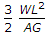# Civil Engineering - Strength of Materials

### Exercise :: Strength of Materials - Section 3

16.

If the stress in each cross-section of a pillar is equal to its working stress, it is called

 A. body of equal B. body of equal section C. body of equal strength D. none of these.

Explanation:

No answer description available for this question. Let us discuss.

17.

A composite member shown in below figure was formed at 25°C and was made of two materials a and b. If the coefficient of thermal expansion of a is more than that of b and the composite member is heated upto 45°C, then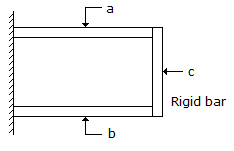A. a will be in tension and b in compression B. both will be in compression C. both will be in tension D. a will be in compression and b in tension.

Explanation:

No answer description available for this question. Let us discuss.

18.

The stress at which extension of a material takes place more quickly as compared to the increase in load, is called

 A. elastic point B. plastic point C. breaking point D. yielding point.

Explanation:

No answer description available for this question. Let us discuss.

19.

In a square beam loaded longitudinally, shear develops

 A. on middle fibre along horizontal plane B. on lower fibre along horizontal plane C. on top fibre along vertical plane D. equally on each fibre along horizontal plane E. none of these.

Explanation:

No answer description available for this question. Let us discuss.

20.

Shear deflection of a cantilever of length L, cross sectional area A and shear modulus G, under a concentrated load W at its free end, is

 A.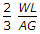B.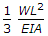C.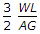D.